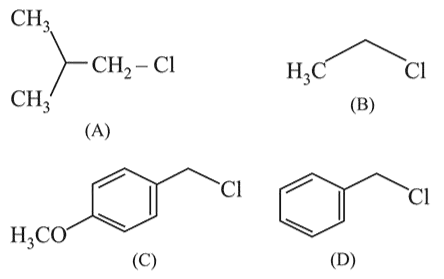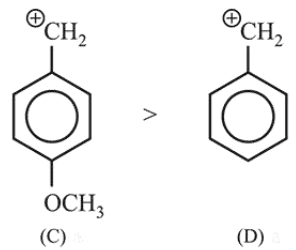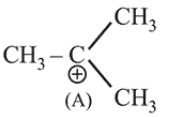# Increasing order of reactivity of the following compounds forQuestion:

Increasing order of reactivity of the following compounds for $S_{N} 1$ substitution is:1. (\mathrm{B})<(\mathrm{C})<(\mathrm{D})<(\mathrm{A})

2. $(B)<(C)<(A)<(D)$

3. $(B)<(A)<(D)<(C)$

4. $(\mathrm{A})<(\mathrm{B})<(\mathrm{D})<(\mathrm{C})$

Correct Option: , 3

Solution:

In $\mathrm{S}_{\mathrm{N}} 1$ reaction carbocation acts as an intermediate.Carbocation produced by $(\mathrm{C})$ is more stable than carbocation produced by (D) due to + I effect of $-\mathrm{OCH}_{3}$ group.

Further in (A) there is formation of tertiary carbocation after rearrangement while (B) is primary carbocation.So, the correct order is $(C)>(D)>(A)>(B)$.